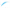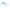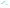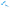# Function Approximation using Data Science Techniques

February 21, 2021

# Regression

I've written about the wonders of Linear Regression before and one of the things I find most amazing about it is that it allows you to approximate a function.

But what does that mean?

In short, take data about two things and estimate a relationship between them.

A classic example is Age and Income.

Suppose you wanted to understand how a person's age correlates to their income. You could take a sample of data and store it in a table, spreadsheet, or even a fancy database somewhere so you could analyze it.

You could then draw a scatter plot (like below) and visualize the relationship between the two attributes and fit (i.e., estimate) the relationship (i.e., the slope of that line). If the relationship was strictly linear, we'd see a scatter plot that looks something like the graph below.A Scatter Plot of Age and Income with a Linear Relationship

But what if it wasn't linear?

What if we knew Age only increased Income to a degree and that the marginal return was decreasing? Well, maybe we'd see a plot like below.A Scatter Plot of Age and Income with a Quadratic Relationship

What if things were a little less intuitive and, after another point, your Income (on average) started to go back up again? We'd see the graph below.A Scatter Plot of Age and Income with a Cubic Relationship

Lastly, what if we saw something that was just plain weird?A Scatter Plot of Age and Income with a Piecewise Linear, Discontinuous Function

This is my favorite example because it shows a piece-wise linear function and, while strange looking, these relationships are very common phenomena in the wild.

Why?

Because often times we are modeling behaviors or decisions by other systems in the world, and those systems, decisions, and behaviors often have weird boundary points/thresholds. In the credit world, you'll often see this because a lender's credit policy systematically rejects applications with a certain set of criteria, which would lead to visualizations identical to this.

## What do we do with all of this information?

This is the fun part!

If you're doing data science and modeling data with lots of features/attributes, you probably don't want to do this for hundreds or thousands of different variables. Instead, you may benefit from finding a way to estimate the univariate relationship algorithmically.

But how?

When you have a bivariate relationship like this you don't want to have to tediously engineer features to estimate the underlying function, rather you'd prefer to have a machine learn (or estimate) the function using supervised learning.

But which algorithm and how do I use it? Well, there are a few options people typically use:

And each has its own unique benefits.

But my personal favorite is MARS. If we used R's earth package we can estimate this function automatically in a few simple lines of code and get the predicted fit below.A Scatter Plot of Age and Income using Function Approximation

And here's the R code to generate it.

``````1library(earth)
2earth.mod <- earth(income ~ age, data = df)
3plotmo(earth.mod)
4print(summary(earth.mod, digits = 2, style = "pmax"))
5dft\$preds <- predict(earth.mod, df)[,1]
``````

Short, sweet, and effective—my favorite combination.

The other algorithms all have pros and cons, and I largely recommend to use each one depending on how smooth or not-smooth the underlying behavior of your data is (and how much you care to really account for it).

Anyways, I hope you liked this post; it was a very enjoyable excuse for me to make some graphs.

Have some feedback? Feel free to let me know!